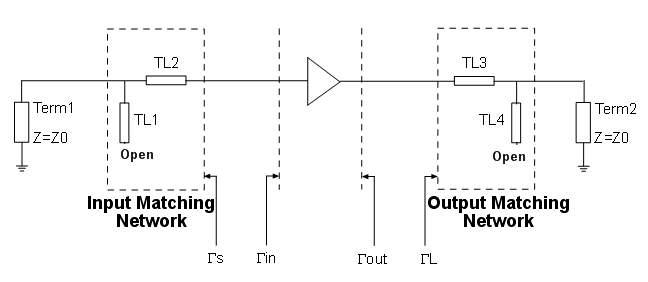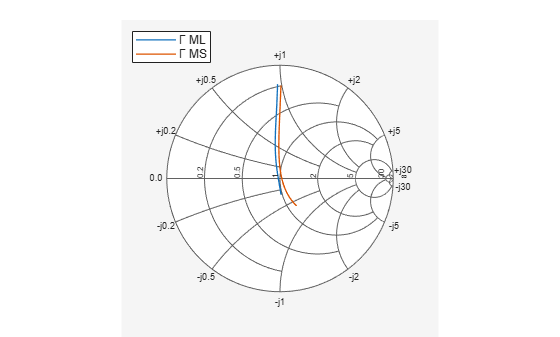# Designing Matching Networks (Part 2: Single Stub Transmission Lines)

This example shows how to use the RF Toolbox to determine the input and output matching networks that maximize power delivered to a 50-Ohm load and system. Designing input and output matching networks is an important part of amplifier design. This example first calculates the reflection factors for simultaneous conjugate match and then determines the placement of a shunt stub in each matching network at a specified frequency. Finally, the example cascades the matching networks with the amplifier and plots the results.

### Create an rfckt.amplifier Object

Create an rfckt.amplifier object to represent the amplifier described by the measured frequency-dependent S-parameter data in the file samplebjt2.s2p. Then, extract the frequency-dependent S-parameter data from the rfckt.amplifier object.

amp = read(rfckt.amplifier,'samplebjt2.s2p'); [sparams,AllFreq] = extract(amp.AnalyzedResult,'S_Parameters');

### Check for Amplifier Stability

Before proceeding with the design, determine the measured frequencies at which the amplifier is unconditionally stable. Use the stabilitymu function to calculate mu and muprime at each frequency. Then, check that the returned values for mu are greater than one. This criteria is a necessary and sufficient condition for unconditional stability. If the amplifier is not unconditionally stable, print out the corresponding frequency value.

[mu,muprime] = stabilitymu(sparams); figure plot(AllFreq/1e9,mu,'--',AllFreq/1e9,muprime,'r') legend('MU',"MU'",'Location','Best') title("Stability Parameters MU and MU'") xlabel('Frequency [GHz]')disp('Measured Frequencies where the amplifier is not unconditionally stable:')
Measured Frequencies where the amplifier is not unconditionally stable: 
fprintf('\tFrequency = %.1e\n',AllFreq(mu<=1))
 Frequency = 1.0e+09 Frequency = 1.1e+09 

For this example, the amplifier is unconditionally stable at all measured frequencies except 1.0 GHz and 1.1 GHz.

### Determine the Source and Load Matching Networks for a Simultaneous Conjugate Match

Begin designing the input and output matching networks by transforming the reflection coefficients for simultaneous conjugate match at the amplifier interfaces into the appropriate source and load admittance. This example uses the following lossless transmission line matching scheme:The design parameters for this single stub matching scheme are the location of the stubs with reference to the amplifier interfaces and the stub lengths. The procedure uses the following design principles:

• The center of the Smith chart represents a normalized source or load immittance.

• Movement along a transmission line is equivalent to traversing a circle centered at the origin of the Smith chart with radius equal to a reflection coefficient magnitude.

• A single transmission line stub can be inserted at the point on a transmission line when its admittance (transmission line) intersects the unity conductance circle. At this location, the stub will negate the transmission line susceptance, resulting in a conductance that equals the load or source terminations.

This example uses the YZ Smith chart because it's easier to add a stub in parallel with a transmission line using this type of Smith chart.

### Calculate and Plot the Complex Load and Source Reflection Coefficients

calculate and plot all complex load and source reflection coefficients for simultaneous conjugate match at all measured frequency data points that are unconditionally stable. These reflection coefficients are measured at the amplifier interfaces.

AllGammaL = calculate(amp,'GammaML','none'); AllGammaS = calculate(amp,'GammaMS','none'); hsm = smithplot([AllGammaL{:} AllGammaS{:}]); hsm.LegendLabels = {'#Gamma ML','#Gamma MS'};### Determine the Load Reflection Coefficient at a Single Frequency

Find the load reflection coefficient, GammaL, for the output matching network at the design frequency 1.9 GHz.

freq = AllFreq(AllFreq == 1.9e9); GammaL = AllGammaL{1}(AllFreq == 1.9e9)
GammaL = -0.0421 + 0.2931i 

### Draw the Constant Magnitude Circle for Load Reflection Coefficient GammaL

Draw a circle that is centered at the normalized admittance Smith chart origin and whose radius equals the magnitude of GammaL. A point on this circle represents the reflection coefficient at a particular location on the transmission line. The reflection coefficient for the transmission line at the amplifier interface is GammaL, while the center of the chart represents the normalized load admittance, y_L. The example uses the circle method to draw all appropriate circles on a Smith chart.

hsm = smithplot; circle(amp,freq,'Gamma',abs(GammaL),hsm); hsm.GridType = 'yz'; hold all plot(0,0,'k.','MarkerSize',16) plot(GammaL,'k.','MarkerSize',16) txtstr = sprintf('\\Gamma_{L}\\fontsize{8}\\bf=\\mid%s\\mid%s^\\circ', ... num2str(abs(GammaL),4),num2str((angle(GammaL)*180/pi),4)); text(real(GammaL),imag(GammaL)+.1,txtstr,'FontSize',10, ... 'FontUnits','normalized'); plot(0,0,'r',0,0,'k.','LineWidth',2,'MarkerSize',16); text(0.05,0,'y_L','FontSize',12,'FontUnits','normalized')

### Draw the Unity Constant Conductance Circle and Find Intersection Points

To determine the stub wavelength (susceptance) and its location with respect to the amplifier load matching interface, plot the normalized unity conductance circle and the constant magnitude circle and figure out where the two circles intersect. Find the points of intersection interactively using the data cursor or analytically using the helper function, find_circle_intersections_helper. This example uses the helper function. The circles intersect at two points. The example uses the third-quadrant point, which is labeled "A". The unity conductance circle is centered at (-.5,0) with radius .5. The constant magnitude circle is centered at (0,0) with radius equal to the magnitude of GammaL.

circle(amp,freq,'G',1,hsm); hsm.ColorOrder(2,:) = [1 0 0]; [~,pt2] = imped_match_find_circle_intersections_helper([0 0], ... abs(GammaL),[-.5 0],.5); GammaMagA = sqrt(pt2(1)^2 + pt2(2)^2); GammaAngA = atan2(pt2(2),pt2(1)); ax = hsm.Parent.CurrentAxes; hold (ax,"on"); plot(ax, pt2(1),pt2(2),'k.','MarkerSize',16); txtstr = sprintf('A=\\mid%s\\mid%s^\\circ',num2str(GammaMagA,4), ... num2str(GammaAngA*180/pi,4)); text(ax, pt2(1),pt2(2)-.07,txtstr,'FontSize',8,'FontUnits','normalized', ... 'FontWeight','Bold') container = hsm.Parent; annotation(container,'textbox','VerticalAlignment','middle',... 'String',{'Unity','Conductance','Circle'},... 'HorizontalAlignment','center','FontSize',8,... 'EdgeColor',[0.04314 0.5176 0.7804],... 'BackgroundColor',[1 1 1],'Position',[0.1403 0.1608 0.1472 0.1396]) annotation(container,'arrow',[0.2786 0.3286],[0.2778 0.3310]) annotation(container,'textbox','VerticalAlignment','middle',... 'String',{'Constant','Magnitude','Circle'},... 'HorizontalAlignment','center','FontSize',8,... 'EdgeColor',[0.04314 0.5176 0.7804],... 'BackgroundColor',[1 1 1],'Position',[0.8107 0.3355 0.1286 0.1454]) annotation(container,'arrow',[0.8179 0.5761],[0.4301 0.4887]);

### Calculate the Stub Location and the Stub Length for the Output Matching Network

The open-circuit stub location in wavelengths from the amplifier load interface is a function of the clockwise angular difference between point "A" and GammaL. When point "A" appears in the third quadrant and GammaL falls in the second quadrant, the stub position in wavelengths is calculated as follows:

StubPositionOut = ((2*pi + GammaAngA) - angle(GammaL))/(4*pi)
StubPositionOut = 0.2147 

The stub value is the amount of susceptance that is required to move the normalized load admittance (the center of the Smith chart) to point "A" on the constant magnitude circle. An open stub transmission line can be used to supply this value of susceptance. Its wavelength is defined by the amount of angular rotation from the open-circuit admittance point on the Smith chart (point "M" on the following figure) to the required susceptance point "N" on the outer edge of the chart. Point "N" is where a constant susceptance circle with a value equal to the susceptance of point "A" intersects the unit circle. In addition, the StubLengthOut formula used below requires "N" to fall in the third or fourth quadrant.GammaA = GammaMagA*exp(1j*GammaAngA); bA = imag((1 - GammaA)/(1 + GammaA)); StubLengthOut = -atan2(-2*bA/(1 + bA^2),(1 - bA^2)/(1 + bA^2))/(4*pi)
StubLengthOut = 0.0883 

### Calculate the Stub Location and the Stub Length for the Input Matching Network

In the previous sections, the example calculated the required lengths and placements, in wavelengths, for the output matching transmission network. Following the same approach, the line lengths for the input matching network are calculated:

GammaS = AllGammaS{1}(AllFreq == 1.9e9)
GammaS = -0.0099 + 0.2501i 
[pt1,pt2] = imped_match_find_circle_intersections_helper([0 0], ... abs(GammaS),[-.5 0],.5); GammaMagA = sqrt(pt2(1)^2 + pt2(2)^2); GammaAngA = atan2(pt2(2),pt2(1)); GammaA = GammaMagA*exp(1j*GammaAngA); bA = imag((1 - GammaA)/(1 + GammaA)); StubPositionIn = ((2*pi + GammaAngA) - angle(GammaS))/(4*pi)
StubPositionIn = 0.2267 
StubLengthIn = -atan2(-2*bA/(1 + bA^2),(1 - bA^2)/(1 + bA^2))/(4*pi)
StubLengthIn = 0.0759 

### Verify the Design

To verify the design, assemble a circuit using 50-Ohm microstrip transmission lines for the matching networks. First, determine if the microstrip line is a suitable choice by analyzing the default microstrip transmission line at a design frequency of 1.9 GHz.

stubTL4 = rfckt.microstrip; analyze(stubTL4,freq); Z0 = stubTL4.Z0;

This characteristic impedance is close to the desired 50-Ohm impedance, so the example can proceed with the design using these microstrip lines.

To calculate the required transmission line lengths in meters for the placement of the stubs, analyze the microstrip to obtain a phase velocity value.

phase_vel = stubTL4.PV;

Use the phase velocity value, which determines the transmission line wavelength and the stub location to set the appropriate transmission line lengths for the two microstrip transmission lines, TL2 and TL3.

TL2 = rfckt.microstrip('LineLength',phase_vel/freq*StubPositionIn); TL3 = rfckt.microstrip('LineLength',phase_vel/freq*StubPositionOut);

Use the phase velocity again to specify stub length and stub mode for each stub.

stubTL1 = rfckt.microstrip('LineLength',phase_vel/freq*StubLengthIn, ... 'StubMode','shunt','Termination','open'); set(stubTL4,'LineLength',phase_vel/freq*StubLengthOut, ... 'StubMode','shunt','Termination','open')

Now cascade the circuit elements and analyze the amplifier with and without the matching networks over the frequency range of 1.5 to 2.3 GHz.

matched_amp = rfckt.cascade('Ckts',{stubTL1,TL2,amp,TL3,stubTL4}); analyze(matched_amp,1.5e9:1e7:2.3e9); analyze(amp,1.5e9:1e7:2.3e9);

To verify the simultaneous conjugate match at the input of the amplifier, plot the S11 parameters in dB for both the matched and unmatched circuits.

clf plot(amp,'S11','dB') hold all hline = plot(matched_amp,'S11','dB'); hline.Color = 'r'; legend('S_{11} - Original Amplifier', 'S_{11} - Matched Amplifier') legend('Location','SouthEast') hold off

To verify the simultaneous conjugate match at the output of the amplifier, plot the S22 parameters in dB for both the matched and unmatched circuits.

plot(amp,'S22','dB') hold all hline = plot(matched_amp,'S22','dB'); hline.Color = 'r'; legend('S_{22} - Original Amplifier', 'S_{22} - Matched Amplifier') legend('Location','SouthEast') hold off

Finally, plot the transducer gain (Gt) and the maximum available gain (Gmag) in dB for the matched circuit.

hlines = plot(matched_amp,'Gt','Gmag','dB'); hlines(2).Color = 'r';

You can see that the transducer gain and the maximum available gain are very close to each other at 1.9 GHz.